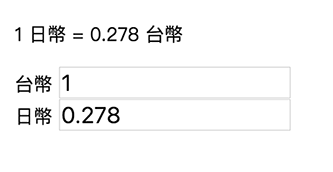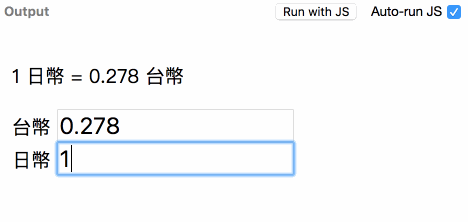# # 1-3 資料加工與邏輯整合

``````<div id="app">
<p> 總金額共 {{ price * quantity }} 元 </p>
<!-- ... 中間略 ... -->
<p> 總金額共 {{ price * quantity }} 元 </p>
<!-- ... 中間略 ... -->
<p> 總金額共 {{ price * quantity }} 元 </p>
</div>
``````
1
2
3
4
5
6
7

## # methods 方法

``````const vm = Vue.createApp({
data () {
return {
price: 100,
quantity: 10
}
},
methods: {
subtotal: function() {
return this.price * this.quantity;
}
}
}).mount('#app');
``````
1
2
3
4
5
6
7
8
9
10
11
12
13

``````<div id="app">
<p> 總金額共 {{ subtotal() }} 元 </p>
<!-- ... 中間略 ... -->
<p> 總金額共 {{ subtotal() }} 元 </p>
<!-- ... 中間略 ... -->
<p> 總金額共 {{ subtotal() }} 元 </p>
</div>
``````
1
2
3
4
5
6
7

``````Vue.createApp({
methods: {
subtotal: () => {
// 小心這裡的 this 由於 arrow function 的關係變成了 window!
return this.price * this.quantity;
}
}
});
``````
1
2
3
4
5
6
7
8

``````Vue.createApp({
methods: {
subtotal () {
// 沒問題！ 這裡的 this 可以對應至 Vue 實體
return this.price * this.quantity;
}
}
});
``````
1
2
3
4
5
6
7
8

(關於 JavaScript 一般函式的各種 `this` 綁定規則可見【0 陷阱！0 誤解！8 天重新認識 JavaScript！】中 "What's "THIS" in JavaScript" 小節，此處不再贅述。)

## # computed 計算屬性

``````const vm = Vue.createApp({
data() {
return {
price: 100,
quantity: 10
}
},
computed: {
subtotal: function() {
return this.price * this.quantity;
}
}
}).mount('#app');
``````
1
2
3
4
5
6
7
8
9
10
11
12
13
``````<div id="app">
<p> 總金額共 {{ subtotal }} 元 </p>
<!-- ... 中間略 ... -->
<p> 總金額共 {{ subtotal }} 元 </p>
<!-- ... 中間略 ... -->
<p> 總金額共 {{ subtotal }} 元 </p>
</div>
``````
1
2
3
4
5
6
7

``````const vm = Vue.createApp({
data () {
return  {
quantity: 100,
price: 100
}
},
computed: {
subtotalComputed: function() {
console.log('computed');
return this.quantity * this.price;
}
},
methods: {
subtotalMethods: function() {
console.log('methods');
return this.quantity * this.price;
}
}
}).mount('#app');
``````
1
2
3
4
5
6
7
8
9
10
11
12
13
14
15
16
17
18
19
20

``````<div id="app">
<p> 總金額共 {{ subtotalComputed }} 元 </p>
<p> 總金額共 {{ subtotalMethods() }} 元 </p>
</div>
``````
1
2
3
4

``````<div id="app">
<p> 總金額共 {{ subtotalComputed }} 元 </p>
<p> 總金額共 {{ subtotalComputed }} 元 </p>
<p> 總金額共 {{ subtotalComputed }} 元 </p>

<p> 總金額共 {{ subtotalMethods() }} 元 </p>
<p> 總金額共 {{ subtotalMethods() }} 元 </p>
<p> 總金額共 {{ subtotalMethods() }} 元 </p>
</div>
``````
1
2
3
4
5
6
7
8
9

`subtotalComputed``subtotalMethods()` 都分別執行了三次， 由於我們在上面的兩個函式裡都寫了 `console.log()`，所以我們打開 console 主控台會看到：

``````computed
methods
methods
methods
``````
1
2
3
4

``````const vm = Vue.createApp({
data () {
return {
message: 'Hello Vue!'
}
},
computed: {
subtotalComputed: function() {
console.log('computed');
return 100 * 100;
}
},
methods: {
subtotalMethods: function() {
console.log('methods');
return 100 * 100;
}
}
}).mount('#app');
``````
1
2
3
4
5
6
7
8
9
10
11
12
13
14
15
16
17
18
19
``````<div id="app">
<p> 總金額共 {{ subtotalComputed }} 元 </p>
<p> 總金額共 {{ subtotalMethods() }} 元 </p>
</div>
``````
1
2
3
4

## # computed / methods 的使用時機比較

``````const vm = Vue.createApp({
data () {
return {
twd: 0.278,
jpy: 1,
}
}
}).mount('#app');
``````
1
2
3
4
5
6
7
8
``````<div id="app">
<p>1 日幣 = 0.278 台幣</p>
<div>台幣 <input type="text" v-model="twd"></div>
<div>日幣 <input type="text" v-model="jpy"></div>
</div>
``````
1
2
3
4
5``````<div id="app">
<p>1 日幣 = 0.278 台幣</p>
<div>台幣 <input type="text" v-model="twd" v-on:input="twd2jpy"></div>
<div>日幣 <input type="text" v-model="jpy" v-on:input="jpy2twd"></div>
</div>
``````
1
2
3
4
5

``````methods: {
twd2jpy () {
this.jpy = Number.parseFloat(Number(this.twd) / 0.278).toFixed(3);
},
jpy2twd () {
this.twd = Number.parseFloat(Number(this.jpy) * 0.278).toFixed(3);
},
}
``````
1
2
3
4
5
6
7
8(程序猿吐血而亡)

``````<div id="app">
<p>1 日幣 = 0.278 台幣</p>
<div>台幣 <input type="text" v-model="twd"></div>
<div>日幣 <input type="text" v-model="jpy"></div>
</div>
``````
1
2
3
4
5

``````const vm = Vue.createApp({
data () {
return {
twd: 0.278
}
},
computed: {
jpy: {
get () {
return Number.parseFloat(Number(this.twd) / 0.278).toFixed(3);
},
set (val) {
this.twd = Number.parseFloat(Number(val) * 0.278).toFixed(3);
}
}
}
}).mount('#app');
``````
1
2
3
4
5
6
7
8
9
10
11
12
13
14
15
16
17

``````usd: {
get () {
return Number.parseFloat(Number(this.twd) / 28.540).toFixed(3);
},
set (val) {
this.twd = Number.parseFloat(Number(val) * 28.540).toFixed(3);
}
}
``````
1
2
3
4
5
6
7
8

## # 補充 - Vue Filters (Vue 2.x)

Vue.js 還有一個與 `methods` 很像的屬性，名為 `filters`，之所以沒有特別列一篇出來介紹， 是因為在實際開發上它使用的機會真的不多，而且在 Vue.js 3.0 這個特性會被移除。

Vue `filters` 的用法與其他 options API 一樣，以物件的形式在實體內定義一個 `filters` 屬性，如：

``````// for Vue 2.x
const vm = new Vue({
data: {
msg: 'hello!'
},
filters: {
capitalize: (value) => {
if (!value) {
return '';
}
value = value.toString();
return value.charAt(0).toUpperCase() + value.slice(1);
},
}
}).\$mount('#app');
``````
1
2
3
4
5
6
7
8
9
10
11
12
13
14
15

``````  <p>{{ msg }}</p>
<p>{{ msg | capitalize }}</p>
``````
1
2

``````hello!
Hello!
``````
1
2

`filters` 會將 `|` (pipe) 符號前面的值當作參數引入，並且回傳對應的內容。看起來跟 `methods` 的作用很像對吧？

``````// for Vue 2.x
const vm = new Vue({
data: {
msg: 'hello!'
},
methods: {
capitalize (value) {
if (!value) {
return '';
}
value = value.toString();
return value.charAt(0).toUpperCase() + value.slice(1);
}
}
}).\$mount('#app');
``````
1
2
3
4
5
6
7
8
9
10
11
12
13
14
15
``````  <p>{{ msg }}</p>
<p>{{ capitalize(msg) }}</p>
``````
1
2

Last Updated: 1/2/2021, 1:30:37 AM